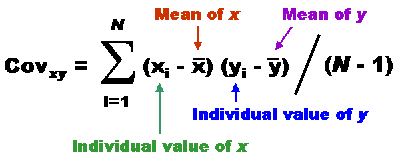# my net house

WAHEGURU….!

## Let’s have fun with Correlation:-O

Ok have some fun first. 😀Whenever you will read any post or paper related to Machine-Learning or Data-Science you will get word ‘Correlation’ many times and how it’s value is important in your model Building.

A simple definition of Correlation: A mutual relationship or connection between two or more things. (that’s layman’s definition and It should be enough most of the times 😉 )

Coefficient of Correlation  is just an integer, From which we understand how two or more things

are related to each-other. As we discussed Coefficient of Correlation is an integer so it could be +ve or -ve and value of correlation decides how two data-sets effect each other.

Following two images tell lot about Correlation and it’s Value.Coefficient of Correlation between range -0.5 to +0.5 is not that valuable by why and how we calculate correlation?What is Covariance ?Now if you still feel that something is really missing we should talk about Variance:

Let’s Remove Co from Covariance.

Variance is Measurement of randomness. So How you would calculate Variance of Data?

Give me data:

Data = [4,5,6,7,12,20]

I will find means and subtract it from each individual- Isn’t that Mean-Deviation ? 😀 OMG!

Have a look at the following Picture:Let’s wait for stuff like:

Coefficient of determination, Probable Error and interpretation.